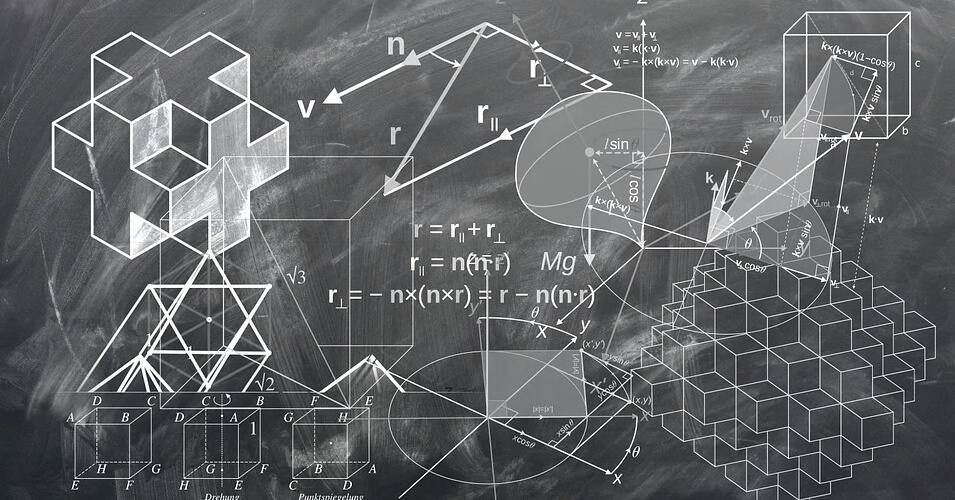• Save

# Volume and Surface Area Questions with AnswersHere I am sharing selective and important multiple-choice volume and surface area questions and answers for competitive exams. Try to solve volume and surface area questions yourself to increase your performance in competitive exams.

## Volume and Surface Area Questions

If you face difficulties to solve these questions, you can visit the surface area and volume problems with solutions to resolve your problems. As well as you should learn how to use formulas of surface area and volume with examples of differential equations in competitive exams.

## Volume And Surface Area

Q :

The slant height of a right circular cone is 15 m and its height is 9 m. The area of its curved surface, is:

(A) 169 π m2

(B) 172 π m2

(C) 165 π m2

(D) 180 π m2

Q :

The height of a cone is 16 cm and radius of its base is 30 cm. If the rate of painting it is Rs.14/cm2, then what will be the total cost in painting the curved surface of cone from outside?

(A) Rs. 42220

(B) Rs. 44880

(C) Rs. 36820

(D) Rs.46540

Q :

The length, breadth and height of a room is 21 metres, 12 metres and 16 metres. What will be the length of the largest rod that can be placed in that room?

(A) 32 metres

(B) 25 metres

(C) 31 metres

(D) 29 metres

Q :

Area of a circle inscribed in a square is 308 cm2.The length of the diagonal of the square is

(A) 16 cm

(B) 28 cm

(C) 24 cm

(D) 22 cm

Q :

The ratio of the areas of two isosceles triangles having the same vertical angle is 1 : 9.The ratio of their heights is -

(A) 1 : 3

(B) 1 : 9

(C) 3 : 1

(D) 1 : 81

Q :

If a chord of length 30 cm is at a distance of 8 cm from the center of the circle, then the length of the chord of the same circle which is at a distance of 15 cm from the center is equal to

(A) 12 cm

(B) 18 cm.

(C) 16 cm

(D) 20 cm.

Q :

The diagonals of a rhombus are 24 cm. and 10 cm. The perimeter (in cm) of this rhombus is-

(A) 54

(B) 68

(C) 52

(D) 54

Q :

Find the volume of the material in a cylindrical tube. The radius of the outer surface being 10 m, the thickness being 0.4 m and the height being 9 m.

(A) $${224 \ {m^{3}}}$$

(B) $${224 \ {m^{3}}}$$

(C) None of these

(D) $${229 \ {m^{3}}}$$

(E) $${218 \ {m^{3}}}$$

Q :

Find the volume of a hemisphere whose radius is equal to the side of an equilateral triangle having area 49√3 cm2 .

(A) 2349.33 cm3

(B) 5749.33 cm3

(C) 4649.33 cm3

(D) 1234.33 cm3

(E) None of these

Q :

If the length of the diagonal of a square is $${ 10\sqrt{2}}$$ cm, then what will be the area of the square?

(A) 200 cm2

(B) 100 cm2

(C) $${ 100\sqrt{2}}$$

(D) 50 cm2